# C++ Array: The Complete Guide

C++ Array is the collection of items stored at contiguous memory locations. The array is the series of elements of the same type placed in contiguous memory locations that can be individually referenced by adding the index to a unique identifier.

Sometimes when writing code, we need to store a lot of data for any calculation, and for that, in the usual way, we have to take that number of variables. Think once, is it logically possible if you are asked to give a summation of 10000 numbers, and you have to take the 10000 variables? The answer is obviously no. To overcome this situation, we have a concept Array.

## C++ Array

C++ Arrays are used to store multiple values in a single variable instead of declaring separate variables for each value. To declare an array, define the variable type, specify the array’s name followed by square brackets and specify the number of elements it should store.

An array is the collection of similar data types, and it is stored in a contiguous memory location. We will understand this concept using a diagram.

Suppose there is 5 number we have to store in an array. So, the array will look like this:-For example, five values of type int can be declared as an array without having to declare five different variables (each with its identifier). Instead, using the array, the five int values are stored in contiguous memory locations, and all five can be accessed using the same identifier with the proper index.

So here, we can see that the Array index always starts from 0, and all values are stored in a contiguous memory location.

There are two types of array:

1. Single Dimensional Array
2. Multi-Dimensional Array

## Single Dimensional Array

### Declaring an Array

If we want to declare an array, the Syntax will follow.

```DataType Array_Name [Size of Array];
```

See the following example.

```int arr;
```

Now, let’s see the following programming example.

```#include<bits/stdc++.h>
using namespace std;
int main()
{
//METHOD 1
int arr1;
arr1=5;
arr1=6;
arr1=7;

//=======METHOD 2=======
int arr2[]={2,5,9,4};

//=======METHOD 3=======
int arr3={7,65,34,33};

//=======METHOD 4========
int n, i;
cout<<"Enter size of array: ";
cin>>n;
int array[n]; //declaring array with size
cout<<"Enter array elements\n";
for(i=0;i<n;i++)
{
cin>>array[i];
}

return 0;
}
```

In the first method, We have declared an array with Array size, and then we store elements using their indices.

In method two, we declared the array without mentioning the size, then stored them in curly braces. In this case, we have to calculate the size later.

In method three, We have declared the array with size, and then we give the elements in curly braces. In both cases, the array index will start from 0.

In method 4, we are taking input from the user during runtime.

## Accessing Array Elements in C++

To access any specific value: For this case, we have to mention the array index, which means which locations value we want to show.

For example, see the below code:

```#include<bits/stdc++.h>
using namespace std;
int main()
{
int n, i;
cout<<"Enter size of array: ";
cin>>n;
int array[n]; //declaring array with size
cout<<"Enter array elements\n";
for(i=0;i<n;i++)
{
cin>>array[i];
}

int id;
cout<<"Enter index of the array\n";
cin>>id;

cout<<"Value at index "<<id<<" is "<<array[id];
return 0;
}
```

See the following output.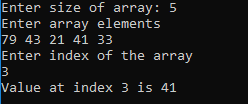To access all the elements: The best way to show all the elements in an array is by using the loop. The below program shows how to access all the elements of an array using a loop:

```#include<bits/stdc++.h>
using namespace std;
int main()
{
int n, i;
cout<<"Enter size of array: ";
cin>>n;
int array[n]; //declaring array with size
cout<<"Enter array elements\n";
for(i=0;i<n;i++)
{
cin>>array[i];
}
cout<<"All entered array values are: \n";
for(i=0;i<n;i++)
{
cout<<array[i]<<" ";
}
return 0;
}
```

See the following Output.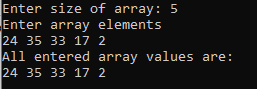## No index bound checking in Array

There is no index of bound checking in Array. C++ throws garbage or unexpected value when the index is out of bounds or range.

The following program shows the index bound checking.

```#include<bits/stdc++.h>
using namespace std;
int main()
{
int n, i;
cout<<"Enter size of array: ";
cin>>n;
int array[n]; //declaring array with size
cout<<"Enter array elements\n";
for(i=0;i<n;i++)
{
cin>>array[i];
}

int id;
cout<<"Enter index of the array\n";
cin>>id; //enter out of bound value

cout<<"Value at index "<<id<<" is "<<array[id];
return 0;
}
```

See the following output.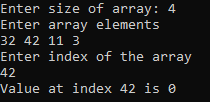## Multi-Dimensional Array

We will learn how to create a 2D array and access those elements.

### Syntax

```DataType Array_name[Size 1][Size 2]
```

See the following code.

`int arr`

Here we have declared a 2D array with 2 rows and 3 columns.

### Storing and accessing elements of 2D array

The 2D array looks something like this diagram.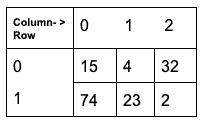On the above diagram, the index of 15 is arr, and the index of 2 is arr means 2nd row and 3rd column.

Note: Row and column index starts from 0.

```#include<bits/stdc++.h>
using namespace std;
int main()
{
int x,y;
cout<<"Enter row number and column number :";
cin>>x>>y;
int arr[x][y],row,col;
cout<<"Enter value of array\n";

for(row=0; row<x; row++)
{
for(col=0; col<y; col++)
{
cin>>arr[row][col];
}
}

cout<<"Value of array are:\n";
for(row=0; row<x; row++)
{
for(col=0; col<y; col++)
{
cout<<arr[row][col]<<" ";
}
cout<<"\n";
}
return 0;
}
```

See the following output.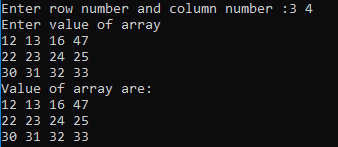So here in the above program, we have declared a 2D array, and then we are storing the values row-wise. The first loop is for row index, and 2nd loop is for column index. Same for accessing the values.

Means, first it will access arr means row 1 and column 1 value, then arr means row 1 and column 2 value and so on.

That’s it for this tutorial.

Categories C++

### 1 thought on “C++ Array: The Complete Guide”

1.Nice I’m also from Gujarat. I also running coding blog 🙂